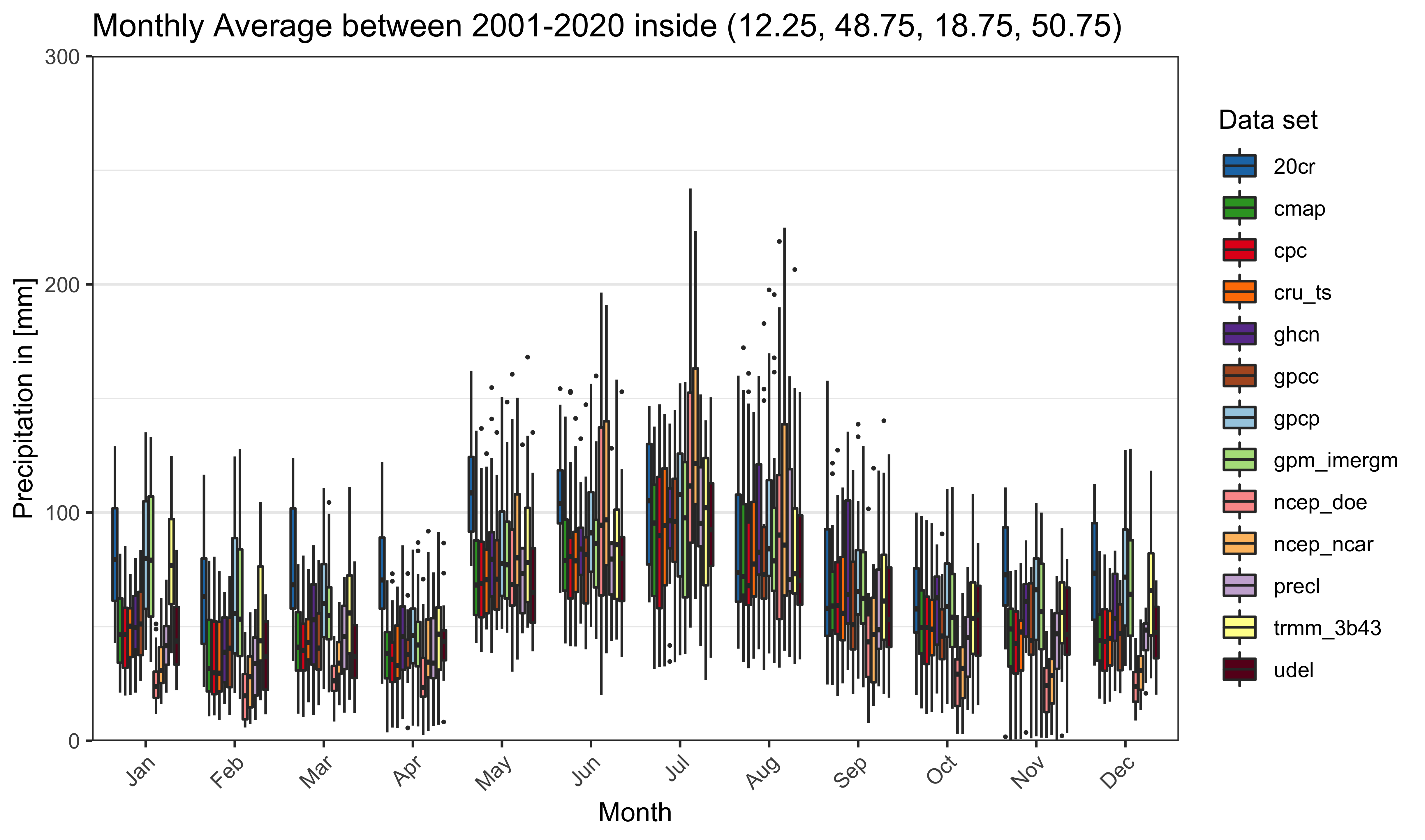# pRecipe: A Multiscale Framework for Data Analysis of Global Precipitation

This is is an introductory presentation of the pRecipe package, as implemented in the study of Vargas Godoy et al. (2022), to assess global water cycle intensification in Czechia.

## Create database

In this study multiple precipitation data sets publicly available were used. Namely: 20CR v3, CMAP, CPC, CRU TS v4.05, GHCN v2, GPCC v2018, GPCP v2.3, GPM IMERGM v06, NCEP/NCAR R1, NCEP/DOE R2, PREC/L, TRMM 3B43 v7, and UDel v5.01. They were all homogenized into monthly 0.5x0.5 data.table stored as a .Rds file per year per data set under ./data/database.

library(pRecipe)
download_data() #(default) download and reformat all the data sets listed above.

## Import data

To import all data or a subset of interest is straightforward:

precip <- import_subset_data(name = "all", start_year = 1836, end_year = 2020,
bbox = c(12.25, 48.75, 18.75, 50.75))

## Crop data

To crop the data the used a shapefile for Czechia

precip <- crop_data(precip, "cz_1km.shp")

## Exploratory Data Analysis

Now we can start exploring the data through different plot options, e.g.:

plot_box(precip[year(Z)>2000 & year(Z)<=2020])